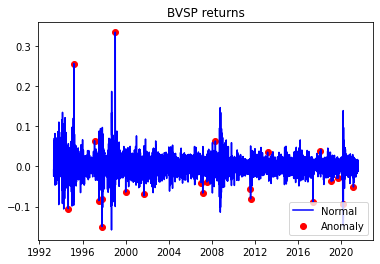# Ibovespa is Brazilian stock market price analysis in python

## What is the Brazilian stock market called?

Brazil's stock market is ^BVSP is the benchmark of 70 stocks.

Analyzing the Ibovespa, the benchmark stock market index of the São Paulo Stock Exchange (B3) in Brazil, using Python involves collecting historical price data, performing data analysis, and creating visualizations to gain insights into the index's performance. Here's a step-by-step guide on how to conduct Ibovespa price analysis in Python:

Import Libraries:

Start by importing the necessary Python libraries for data manipulation, analysis, and visualization. Commonly used libraries include pandas, numpy, and matplotlib. You may also need yfinance or another financial data API to fetch historical data:

Python

Copy code

import pandas as pd

import numpy as np

import matplotlib.pyplot as plt

import yfinance as yf

Data Retrieval:

Use the yfinance library to fetch historical Ibovespa data. Specify the start and end dates for the data you want to analyze:

Python

Copy code

Data Exploration:

Explore the fetched data to understand its structure and contents. You can use functions like head(), tail(), describe(), and info():

Python

Copy code

Data Visualization:

Create visualizations to analyze the historical performance of the Ibovespa. Common visualizations include line charts to visualize the index's price movements:

Python

Copy code

plt.figure(figsize=(12, 6))

plt.title('Ibovespa Index Price')

plt.xlabel('Date')

plt.ylabel('Price')

plt.legend()

plt.show()

Technical Analysis (Optional):

You can perform technical analysis by calculating and visualizing technical indicators like moving averages, relative strength index (RSI), and MACD. Popular Python libraries like ta-lib can help with these calculations.

Statistical Analysis (Optional):

Conduct statistical analysis to calculate summary statistics, volatility measures, and correlations with other assets. You can use Numpy and Pandas for these calculations.

Sentiment Analysis (Optional):

Consider incorporating sentiment analysis of news articles or social media data related to the Brazilian stock market to understand market sentiment's impact on Ibovespa.

Fundamental Analysis (Optional):

Analyze fundamental factors affecting the Brazilian economy, such as GDP growth, inflation rates, and interest rates, which can influence Ibovespa's performance.

Prediction and Forecasting (Optional):

You can use time series forecasting techniques like ARIMA or machine learning models to make predictions about future Ibovespa movements.

Risk Management and Decision Making:

Based on your analysis, formulate investment strategies, set risk management parameters, and make informed investment decisions regarding Ibovespa or Brazilian stocks.

Keep your analysis up to date with the latest data to adapt to changing market conditions and make timely decisions.

Remember that investing in stock markets carries risks, and it's crucial to do thorough research, consider the political and economic climate, and potentially consult with financial experts before making any investment decisions based on your analysis of the Ibovespa or any other stock index.

IBOVESPA (^BVSP) is Brl means brazil

<matplotlib.legend.Legend at 0x7ff576dc1550>
<matplotlib.legend.Legend at 0x7ff576dc1550>#### Need some code in Python

import yfinance as yf

import numpy as np

import pandas as pd

import seaborn as sns

import scipy.stats as scs

import statsmodels.api as sm

import statsmodels.tsa.api as smt

import matplotlib.pyplot as plt

### How to collect data in Python?

start='1985-01-01',

end='2021-07-29',

progress=False)

## Find simple and log return

df[['simple_rtn','log_rtn']].tail(20)

Get simple and log the return

Define Relise volatility code in Python

def realized_volatility(x):

return np.sqrt(np.sum(x**2))

df_rv = df.groupby(pd.Grouper(freq='M')).apply(realized_volatility)

df_rv.rename(columns={'log_rtn': 'rv'}, inplace=True)

df_rv.rv = df_rv.rv * np.sqrt(12)

fig, ax = plt.subplots(2, 1, sharex=True)

ax.plot(df)

ax.plot(df_rv)

Plot relise volatility

## How to plot simple, log returns in Python?

fig, ax = plt.subplots(3, 1, figsize=(24, 20), sharex=True)

ax.set(title = 'BVSP time series',

ylabel = 'Stock price ($)') df.simple_rtn.plot(ax=ax) ax.set(ylabel = 'Simple returns (%)') df.log_rtn.plot(ax=ax) ax.set(xlabel = 'Date', ylabel = 'Log returns (%)') Log return fig, ax = plt.subplots() ax.plot(df_outliers.index, df_outliers.simple_rtn, color='blue', label='Normal') ax.scatter(outliers.index, outliers.simple_rtn, color='red', label='Anomaly') ax.set_title("BVSP returns") ax.legend(loc='lower right') Outliers simple return plot ### Correction between Ibovespa and Vix ax = sns.regplot(x='log_rtn', y='vol_rtn', data=df, line_kws={'color': 'red'}) ax.set(title=f'BVSP vs. VIX ($\\rho\$ = {corr_coeff:.2f})',

ylabel='VIX log returns',

xlabel='BVSP log returns')

Plot correlation between BVSP and VIX

New updated IBOVESPA

Learn PYTHON